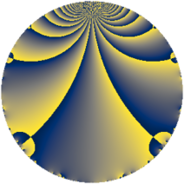# Properties

 Label 285.2.aLevel $285$ Weight $2$ Character orbit 285.a Rep. character $\chi_{285}(1,\cdot)$ Character field $\Q$ Dimension $11$ Newform subspaces $7$ Sturm bound $80$ Trace bound $5$

# Related objects

## Defining parameters

 Level: $$N$$ $$=$$ $$285 = 3 \cdot 5 \cdot 19$$ Weight: $$k$$ $$=$$ $$2$$ Character orbit: $$[\chi]$$ $$=$$ 285.a (trivial) Character field: $$\Q$$ Newform subspaces: $$7$$ Sturm bound: $$80$$ Trace bound: $$5$$ Distinguishing $$T_p$$: $$2$$, $$7$$

## Dimensions

The following table gives the dimensions of various subspaces of $$M_{2}(\Gamma_0(285))$$.

Total New Old
Modular forms 44 11 33
Cusp forms 37 11 26
Eisenstein series 7 0 7

The following table gives the dimensions of the cuspidal new subspaces with specified eigenvalues for the Atkin-Lehner operators and the Fricke involution.

$$3$$$$5$$$$19$$FrickeDim
$$+$$$$+$$$$+$$$+$$$1$$
$$+$$$$+$$$$-$$$-$$$2$$
$$+$$$$-$$$$+$$$-$$$3$$
$$-$$$$+$$$$+$$$-$$$2$$
$$-$$$$+$$$$-$$$+$$$1$$
$$-$$$$-$$$$-$$$-$$$2$$
Plus space$$+$$$$2$$
Minus space$$-$$$$9$$

## Trace form

 $$11 q + 5 q^{2} - q^{3} + 13 q^{4} - q^{5} - 3 q^{6} + 9 q^{8} + 11 q^{9} + O(q^{10})$$ $$11 q + 5 q^{2} - q^{3} + 13 q^{4} - q^{5} - 3 q^{6} + 9 q^{8} + 11 q^{9} - 3 q^{10} + 12 q^{11} - 7 q^{12} - 6 q^{13} - q^{15} + 21 q^{16} - 10 q^{17} + 5 q^{18} - q^{19} + 9 q^{20} - 8 q^{21} + 4 q^{22} + 9 q^{24} + 11 q^{25} - 26 q^{26} - q^{27} - 8 q^{28} + 10 q^{29} + q^{30} - 7 q^{32} - 4 q^{33} - 6 q^{34} + 13 q^{36} + 2 q^{37} - 3 q^{38} - 6 q^{39} - 15 q^{40} - 18 q^{41} + 8 q^{42} + 20 q^{43} + 12 q^{44} - q^{45} - 32 q^{46} + 8 q^{47} - 31 q^{48} - 13 q^{49} + 5 q^{50} + 14 q^{51} - 42 q^{52} + 10 q^{53} - 3 q^{54} + 20 q^{55} - 48 q^{56} + 3 q^{57} - 42 q^{58} + 52 q^{59} - 7 q^{60} - 22 q^{61} - 56 q^{62} + 21 q^{64} - 6 q^{65} - 20 q^{66} + 12 q^{67} - 70 q^{68} - 8 q^{69} - 8 q^{70} + 24 q^{71} + 9 q^{72} - 10 q^{73} - 26 q^{74} - q^{75} - 7 q^{76} + 8 q^{77} - 2 q^{78} + q^{80} + 11 q^{81} + 2 q^{82} + 4 q^{83} - 8 q^{84} - 2 q^{85} + 4 q^{86} + 10 q^{87} + 20 q^{88} - 18 q^{89} - 3 q^{90} + 48 q^{91} + 64 q^{92} - 16 q^{93} + 56 q^{94} - q^{95} - 15 q^{96} - 58 q^{97} + 29 q^{98} + 12 q^{99} + O(q^{100})$$

## Decomposition of $$S_{2}^{\mathrm{new}}(\Gamma_0(285))$$ into newform subspaces

Label Dim $A$ Field CM Traces A-L signs $q$-expansion
$a_{2}$ $a_{3}$ $a_{5}$ $a_{7}$ 3 5 19
285.2.a.a $1$ $2.276$ $$\Q$$ None $$-1$$ $$1$$ $$-1$$ $$-2$$ $-$ $+$ $-$ $$q-q^{2}+q^{3}-q^{4}-q^{5}-q^{6}-2q^{7}+\cdots$$
285.2.a.b $1$ $2.276$ $$\Q$$ None $$1$$ $$-1$$ $$-1$$ $$-2$$ $+$ $+$ $+$ $$q+q^{2}-q^{3}-q^{4}-q^{5}-q^{6}-2q^{7}+\cdots$$
285.2.a.c $1$ $2.276$ $$\Q$$ None $$1$$ $$-1$$ $$1$$ $$4$$ $+$ $-$ $+$ $$q+q^{2}-q^{3}-q^{4}+q^{5}-q^{6}+4q^{7}+\cdots$$
285.2.a.d $2$ $2.276$ $$\Q(\sqrt{7})$$ None $$0$$ $$-2$$ $$2$$ $$-2$$ $+$ $-$ $+$ $$q+\beta q^{2}-q^{3}+5q^{4}+q^{5}-\beta q^{6}+(-1+\cdots)q^{7}+\cdots$$
285.2.a.e $2$ $2.276$ $$\Q(\sqrt{3})$$ None $$0$$ $$2$$ $$2$$ $$-2$$ $-$ $-$ $-$ $$q+\beta q^{2}+q^{3}+q^{4}+q^{5}+\beta q^{6}+(-1+\cdots)q^{7}+\cdots$$
285.2.a.f $2$ $2.276$ $$\Q(\sqrt{2})$$ None $$2$$ $$-2$$ $$-2$$ $$4$$ $+$ $+$ $-$ $$q+(1+\beta )q^{2}-q^{3}+(1+2\beta )q^{4}-q^{5}+\cdots$$
285.2.a.g $2$ $2.276$ $$\Q(\sqrt{2})$$ None $$2$$ $$2$$ $$-2$$ $$0$$ $-$ $+$ $+$ $$q+(1+\beta )q^{2}+q^{3}+(1+2\beta )q^{4}-q^{5}+\cdots$$

## Decomposition of $$S_{2}^{\mathrm{old}}(\Gamma_0(285))$$ into lower level spaces

$$S_{2}^{\mathrm{old}}(\Gamma_0(285)) \cong$$ $$S_{2}^{\mathrm{new}}(\Gamma_0(15))$$$$^{\oplus 2}$$$$\oplus$$$$S_{2}^{\mathrm{new}}(\Gamma_0(19))$$$$^{\oplus 4}$$$$\oplus$$$$S_{2}^{\mathrm{new}}(\Gamma_0(57))$$$$^{\oplus 2}$$$$\oplus$$$$S_{2}^{\mathrm{new}}(\Gamma_0(95))$$$$^{\oplus 2}$$